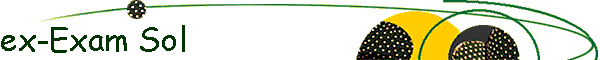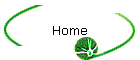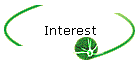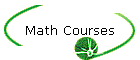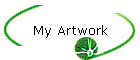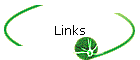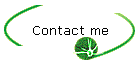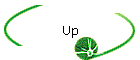College Algebra and Trigonometry II Solutions of selected old-exams problems. Chapter 4: Exponential and Logarithmic Functions. Chapter 5: Trigonometric Functions.Chapter 6: Trig. Identities and Equations.Chapter 7: Applications of Trig (Vectors).Chapter 8: Topics in Analytic Geometry.Chapter 9: Systems of Equations. Chapter 10: Matrices.Escher (A. Farhat 2004)